comm.MIMOChannel

Filter input signal through MIMO multipath fading channel

Description

The comm.MIMOChannel System object™ filters an input signal through a multiple-input/multiple-output (MIMO) multipath fading channel. This object models Rayleigh and Rician fading and employs the Kronecker model for modeling the spatial correlation between the links. For processing details, see the Algorithms section.

To filter an input signal through a MIMO multipath fading channel:

1. Create the comm.MIMOChannel object and set its properties.

2. Call the object with arguments, as if it were a function.

Creation

Description

mimochannel = comm.MIMOChannel creates a MIMO frequency-selective or frequency-flat fading channel System object.

example

mimochannel = comm.MIMOChannel(Name,Value) sets properties using one or more name-value arguments. For example, 'SampleRate',2 sets the input signal sample rate to 2.

Properties

expand all

Unless otherwise indicated, properties are nontunable, which means you cannot change their values after calling the object. Objects lock when you call them, and the release function unlocks them.

If a property is tunable, you can change its value at any time.

Input signal sample rate in hertz, specified as a positive scalar.

Data Types: double

Discrete path delay in seconds, specified as a scalar or row vector.

• When you set PathDelays to a scalar, the MIMO channel is frequency flat.

• When you set PathDelays to a vector, the MIMO channel is frequency selective.

The PathDelays and AveragePathGains properties must be the same length.

Data Types: double

Average path gains in decibels, specified as a scalar or row vector. The AveragePathGains and PathDelays properties must be the same length.

Data Types: double

Normalize path gains, specified as one of these logical values:

• 1 (true) — The fading processes are normalized so that the total power of the path gains, averaged over time, is 0 dB.

• 0 (false) — The total power of the path gains is not normalized.

The AveragePathGains property specifies the average powers of the path gains.

Data Types: logical

Fading distribution to use for the channel, specified as 'Rayleigh' or 'Rician'.

Data Types: char | string

K-factor of a Rician fading channel, specified as a positive scalar or a 1-by-NP vector of nonnegative values. NP is the number of discrete path delays specified by the PathDelays property.

• When you set KFactor to a scalar, the first discrete path is a Rician fading process with a Rician K-factor of KFactor. Any remaining discrete paths are independent Rayleigh fading processes.

• When you set KFactor to a vector, the discrete path corresponding to a positive element of the KFactor vector is a Rician fading process with a Rician K-factor specified by that element. The discrete path corresponding to any zero-valued elements of the KFactor vector are Rayleigh fading processes. At least one element must be nonzero.

Dependencies

To enable this property, set the FadingDistribution property to 'Rician'.

Data Types: double

Doppler shifts for the line-of-sight components of the multipath Rician fading channel, specified as a scalar or row vector. Units are in hertz. This property must be the same size as the KFactor property.

• When you set DirectPathDopplerShift to a scalar, the value represents the line-of-sight component Doppler shift of the first discrete path. This path exhibits a Rician fading process.

• When you set DirectPathDopplerShift to a row vector, the discrete path corresponding to a positive element of the KFactor vector is a Rician fading process. The corresponding element of DirectPathDopplerShift specifies the line-of-sight component for the Doppler shift of that discrete path.

Dependencies

To enable this property, set the FadingDistribution property to 'Rician'.

Data Types: double

Initial phases for the line-of-sight components of the multipath Rician fading channel, specified as a scalar or row vector. Units are in radians. This property must be the same size as the KFactor property value.

• When you set DirectPathInitialPhase to a scalar, the value represents the line-of-sight component initial phase of the first discrete path. This path exhibits a Rician fading process.

• When you set DirectPathInitialPhase to a row vector, the discrete path corresponding to a positive element of the KFactor vector is a Rician fading process. The corresponding element of DirectPathInitialPhase specifies the line-of-sight component for the initial phase of that discrete path.

Dependencies

To enable this property, set the FadingDistribution property to 'Rician'.

Data Types: double

Maximum Doppler shift for all channel paths, specified as a nonnegative scalar. Units are in hertz.

The maximum Doppler shift limit applies to each channel path. When you set this property to 0, the channel remains static for the entire input. You can use the reset object function to generate a new channel realization. The MaximumDopplerShift property value must be smaller than SampleRate/10/fc for each path, where fc is the cutoff frequency factor of the path. For most Doppler spectrum types, the value of fc is 1. For Gaussian and bi-Gaussian Doppler spectrum types, fc is dependent on the Doppler spectrum structure fields. For more details about how fc is defined, see the Cutoff Frequency Factor section.

Data Types: double

Doppler spectrum shape for all channel paths, specified as a Doppler spectrum structure or a 1-by-NP cell array of Doppler spectrum structures. These Doppler spectrum structures must be outputs of the form returned from the doppler function. NP is the number of discrete path delays specified by the PathDelays property. The MaximumDopplerShift property defines the maximum Doppler shift value that the DopplerSpectrum property permits when you specify the Doppler spectrum.

• When you set DopplerSpectrum to a single Doppler spectrum structure, all paths have the same specified Doppler spectrum.

• When you set DopplerSpectrum to a cell array of Doppler spectrum structures, each path has the Doppler spectrum specified by the corresponding structure in the cell array.

Specify options for the spectrum type by using the specType input to the doppler function. If you set the FadingTechnique property to 'Sum of sinusoids', you must set DopplerSpectrum to doppler('Jakes').

Dependencies

To enable this property, set the MaximumDopplerShift property to a positive scalar.

Data Types: struct | cell

Spatial correlation specification, specified as 'Separate Tx Rx', 'None', or 'Combined'.

• Choose 'Spatial Tx Rx' to separately specify the transmit and receive spatial correlation matrices from which the number of transmit antennas (NT) and number of receive antennas (NR) are derived.

• Choose 'None' to specify the number of transmit and receive antennas.

• Choose 'Combined' to specify a single correlation matrix for the whole channel from which the product of NT and NR is derived.

Data Types: char | string

Number of transmit antennas, specified as a positive integer.

Dependencies

To enable this property, set the SpatialCorrelationSpecification property to 'None' or 'Combined'.

Data Types: double

Number of receive antennas, specified as a positive integer.

Dependencies

To enable this property, set the SpatialCorrelationSpecification property to 'None' or 'Combined'.

Data Types: double

Spatial correlation of the transmitter, specified as an NT-by-NT matrix or NT-by-NT-by-NP array. NT is the number of transmit antennas. NP is the number of discrete path delays specified by the PathDelays property.

• If you set PathDelays to a scalar, the channel is frequency flat and TransmitCorrelationMatrix must be an NT-by-NT Hermitian matrix. The magnitude of any off-diagonal element must be no larger than the geometric mean of the two corresponding diagonal elements.

• If you set PathDelays to a vector, the channel is frequency selective and you can specify TransmitCorrelationMatrix as one of these options:

• An NT-by-NT matrix. In this case, each path has the same transmit spatial correlation matrix.

• An NT-by-NT-by-NP array. In this case, each path has its own specified transmit spatial correlation matrix.

Dependencies

To enable this property, set the SpatialCorrelationSpecification property to 'Separate Tx Rx'.

Data Types: double
Complex Number Support: Yes

Spatial correlation of the receiver, specified as an NR-by-NR matrix or NR-by-NR-by-NP array. NR is the number of receive antennas. NP is the number of discrete path delays specified by the PathDelays property.

• If you set PathDelays to a scalar, the channel is frequency flat, and ReceiveCorrelationMatrix must be an NR-by-NR Hermitian matrix. The magnitude of any off-diagonal element must be no larger than the geometric mean of the two corresponding diagonal elements.

• If you set PathDelays to a vector, the channel is frequency selective and you can specify ReceiveCorrelationMatrix as one of these options:

• An NR-by-NR matrix. In this case, each path has the same receive spatial correlation matrix.

• An NR-by-NR-by-NP array. In this case, each path has its own specified receive spatial correlation matrix.

Dependencies

To enable this property, set the SpatialCorrelationSpecification property to 'Separate Tx Rx'.

Data Types: double
Complex Number Support: Yes

Combined spatial correlation matrix, specified as an NTR-by-NTR matrix or NTR-by-NTR-by-NP array. NTR = (NTNR), and NP is the number of discrete delay paths (the length of the PathDelays property).

• If PathDelays is a scalar, the channel is frequency flat, and SpatialCorrelationMatrix must be an NTR-by-NTR Hermitian matrix. The magnitude of any off-diagonal element must be no larger than the geometric mean of the two corresponding diagonal elements.

• If you set PathDelays to a vector, the channel is frequency selective and you can specify SpatialCorrelationMatrix as one of these options:

• An NTR-by-NTR matrix. In this case, each path has the same combined spatial correlation matrix.

• An NTR-by-NTR-by-NP array. In this case, each path has its own specified combined spatial correlation matrix.

Dependencies

To enable this property, set the SpatialCorrelationSpecification property to 'Combined'.

Data Types: double
Complex Number Support: Yes

Antenna selection scheme, specified as 'Off', 'Tx', 'Rx', or 'Tx and Rx'.

Tx represents transmit antennas, and Rx represents receive antennas. When you configure any antenna selection other than the default setting, the object requires one or more inputs to specify which antennas are selected for signal transmission. For more information, see Antenna Selection.

Data Types: char | string

Normalize channel outputs, specified as one of these logical values:

• 1 (true) — The channel outputs are normalized by the number of receive antennas.

• 0 (false) — The channel outputs are not normalized.

Data Types: logical

Channel filtering, specified as one of these logical values:

• 1 (true) — The channel accepts an input signal and produces a filtered output signal.

• 0 (false) — The object does not accept an input signal, produces no filtered output signal, and outputs only channel path gains. You must specify the duration of the fading process by using the NumSamples property.

Data Types: logical

Output channel path gains, specified as a logical 0 (false) or 1 (true). Set this property to true to output the channel path gains of the underlying fading process.

Dependencies

To enable this property, set the ChannelFiltering property to true.

Data Types: logical

Number of samples used for the duration of the fading process, specified as a nonnegative integer.

Tunable: Yes

Dependencies

To enable this property, set the ChannelFiltering property to false.

Data Types: double

Path gain output data type, specified as 'double' or 'single'.

Dependencies

To enable this property, set the ChannelFiltering property to false.

Data Types: char | string

Channel model fading technique, specified as 'Filtered Gaussian noise' or 'Sum of sinusoids'.

Data Types: char | string

Number of sinusoids used to model the fading process, specified as a positive integer.

Dependencies

To enable this property, set the FadingTechnique property to 'Sum of sinusoids'.

Data Types: double

Source to control the start time of the fading process, specified as 'Property' or 'Input port'.

• When you set InitialTimeSource to 'Property', set the initial time offset by using the InitialTime property.

• When you set InitialTimeSource to 'Input port', specify the start time of the fading process by using the inittime input argument. The input value can change in consecutive calls to the object.

Dependencies

To enable this property, set the FadingTechnique property to 'Sum of sinusoids'.

Data Types: char | string

Initial time offset for the fading model in seconds, specified as a nonnegative scalar.

InitialTime must be greater than the end time of the last frame. When mod(InitialTime/SampleRate is nonzero, the object rounds the initial time offset up to the nearest sample position.

Dependencies

To enable this property, set the FadingTechnique property to 'Sum of sinusoids' and the InitialTimeSource property to 'Property'.

Data Types: double

Source of the random number stream, specified as 'Global stream' or 'mt19937ar with seed'.

• When you specify 'Global stream', the object uses the current global random number stream for normally distributed random number generation. In this case, the reset object function resets only the filters.

• When you specify 'mt19937ar with seed', the object uses the mt19937ar algorithm for normally distributed random number generation. In this case, the reset object function resets the filters and reinitializes the random number stream to the value of the Seed property.

Data Types: char | string

Initial seed of the mt19937ar random number stream, specified as a nonnegative integer. When you call the reset object function, it reinitializes the mt19937ar random number stream to the Seed value.

Dependencies

To enable this property, set the RandomStream property to 'mt19937ar with seed'.

Data Types: double

Channel visualization, specified as 'Off', 'Impulse response', 'Frequency response', 'Impulse and frequency responses', or 'Doppler spectrum'. When you set the channel visualization to a value other than 'Off', the selected channel characteristics, such as impulse response or Doppler spectrum, display in a separate window. For more information, see Channel Visualization.

Dependencies

To enable this property, set the FadingTechnique property to 'Filtered Gaussian noise'.

Data Types: char | string

Transmit-receive antenna pair to display, specified as a two element row vector. The first element corresponds to the desired transmit antenna, and the second element corresponds to the desired receive antenna. Only a single pair can be displayed.

Dependencies

To enable this property, set the Visualization property to 'Impulse response', 'Frequency response', 'Doppler spectrum', or 'Impulse and frequency responses'.

Data Types: double

Path for which the Doppler spectrum is displayed, specified as an integer in the range [1, NP]. NP is the number of discrete path delays specified by the PathDelays property. Use this property to select the discrete path used in constructing a Doppler spectrum plot.

Dependencies

To enable this property, set the Visualization property to 'Doppler spectrum'.

Data Types: double

Percentage of samples to display, specified as '25%', '10%', '50%', or '100%'. Increasing the percentage improves display accuracy at the expense of simulation speed.

Dependencies

To enable this property, set the Visualization property to 'Impulse response', 'Frequency response', or 'Impulse and frequency responses'.

Data Types: char | string

Usage

Description

example

y = mimochannel(x) filters the input signal x through the MIMO fading channel and returns the result in y.

To enable this syntax, set the ChannelFiltering property to true.

y = mimochannel(x,seltx) filters the input signal through the MIMO fading channel by using the transmit antennas specified by seltx.

To enable this syntax set the AntennaSelection property to 'Tx'.

For example, this code shows how to select the first and third transmit antenna index as active.

mimochannel = comm.MIMOChannel('AntennaSelection','Tx');
seltx = [1 0 1];
...
y = mimochannel(x,seltx);

y = mimochannel(x,selrx) filters the input signal through the MIMO fading channel by using the receive antennas selected by selrx.

To enable this syntax set the AntennaSelection property to 'Rx'.

For example, this code shows how to select the second receive antenna index as active.

mimochannel = comm.MIMOChannel('AntennaSelection','Rx');
selrx = [0 1];
...
y = mimochannel(x,selrx);

example

y = mimochannel(x,seltx,selrx) filters the input signal through the MIMO fading channel by using the transmit and receive antennas selected by seltx and selrx.

To enable this syntax set the AntennaSelection property to 'Tx and Rx'.

For example, this code shows how to select the first and second transmit antenna and the second receive antenna as active.

mimochannel = comm.MIMOChannel( ...
'AntennaSelection','Tx and Rx');
seltx = [1 1];
selrx = [0 1];
...
y = mimochannel(x,selrx);

example

y = mimochannel(___,inittime) specifies a start time for the fading process in addition to an input argument combination from any of the previous syntaxes.

To enable this syntax, also set the FadingTechnique property to 'Sum of sinusoids' and the InitialTimeSource property to 'Input port'.

example

[y,pathgains] = mimochannel(___) also returns the MIMO channel path gains for antenna selection schemes using any of the input argument combinations in the previous syntaxes.

pathgains = mimochannel() returns the channel path gains of the underlying fading process. In this case, the channel requires no input signal and acts as a source of path gains.

To enable this syntax, set the ChannelFiltering property to false.

pathgains = mimochannel(seltx) returns the channel path gains of the underlying fading process by using the transmit antennas specified by seltx.

To enable this syntax set ChannelFiltering property to false and the AntennaSelection property to 'Tx'.

pathgains = mimochannel(selrx) returns the channel path gains of the underlying fading process by using the transmit antennas specified by selrx.

To enable this syntax set the ChannelFiltering property to false and the AntennaSelection property to 'Rx'.

pathgains = mimochannel(seltx,selrx) returns the channel path gains of the underlying fading process by using the transmit and receive antennas selected by seltx and selrx.

To enable this syntax set the ChannelFiltering property to false and the AntennaSelection property to 'Tx and Rx'.

example

pathgains = mimochannel(___,inittime) specifies a start time for the fading process in addition to an input argument combination from any of the previous syntaxes.

To enable this syntax, also set the FadingTechnique property to 'Sum of sinusoids' and the InitialTimeSource property to 'Input port'.

Input Arguments

expand all

Input signal, specified as a scalar, an NS-element column vector, or an NS-by-NT or NS-by-NST matrix.

• NS is the number of samples.

• NT is the number of transmit antennas and is determined by the TransmitCorrelationMatrix or NumTransmitAntennas property values.

• NST is the number of selected transmit antennas and is determined by the number of elements that are set to 1 in the vector provided by the seltx input.

Data Types: single | double
Complex Number Support: Yes

Select active transmit antennas, specified as a 1-by-NT binary-vaued vector. NT is the number of transmit antennas. Elements set to 1 identify selected antenna indices, and the elements set to 0 identify nonselected antenna indices.

Data Types: single | double

Select active receive antennas, specified as a 1-by-NR binary-valued vector. NR is the number of receive antennas. Elements set to 1 identify selected antenna indices, and the elements set to 0 identify nonselected antenna indices.

Data Types: single | double

Initial time offset for the fading model in seconds, specified as a nonnegative scalar.

When mod(inittime/SampleRate, the initial time offset is rounded up to the nearest sample position.

Data Types: single | double

Output Arguments

expand all

Output signal, returned as an NS-by-NR or NS-by-NSR matrix.

• NS is the number of samples.

• NR is the number of receive antennas and is determined by the ReceiveCorrelationMatrix or NumReceiveAntennas property values.

• NSR is the number of selected receive antennas and is determined by the number of elements that are set to 1 in the vector provided by the selrx input.

Output path gains, returned as an NS-by-NP-by-NT-by-NR array with NaN values for the unselected transmit-receive antenna pairs. pathgains contains complex values.

• NS is the number of samples.

• NP is the number of discrete path delays specified by the PathDelays property.

• NT is the number of transmit antennas.

• NR is the number of receive antennas.

When you set the ChannelFiltering property to false, the data type of this output has the same precision as the input signal x. When you set the ChannelFiltering property to true, the data type of this output is specified by the OutputDataType property.

Object Functions

To use an object function, specify the System object as the first input argument. For example, to release system resources of a System object named obj, use this syntax:

release(obj)

expand all

 step Run System object algorithm release Release resources and allow changes to System object property values and input characteristics reset Reset internal states of System object

Note

• If you set the RandomStream property of the object to 'Global stream', the reset object function resets the filters only.

• If you set RandomStream to 'mt19937ar with seed', the reset object function resets the filters and also reinitializes the random number stream to the value of the Seed property.

Examples

collapse all

Create a 4-by-2 MIMO channel by using the MIMO channel System object. Modulate and spatially encode data, and then pass the data through the channel.

Generate QPSK-modulated data.

data = randi([0 3],1000,1);
modData = pskmod(data,4,pi/4);

Create an orthogonal space-time block encoder System object to encode the modulated data into four spatially separated streams. Then, encode the data.

ostbc = comm.OSTBCEncoder( ...
'NumTransmitAntennas',4, ...
'SymbolRate',1/2);
txSig = ostbc(modData);

Create a MIMO channel System object, using name-value pairs to set the properties. The channel consists of two paths, each with a maximum Doppler shift of 5 Hz. Set the SpatialCorrelationSpecification property to 'None', which requires that you specify the number of transmit and receive antennas. Specify four transmit antennas and two receive antennas.

mimochannel = comm.MIMOChannel( ...
'SampleRate',1000, ...
'PathDelays',[0 2e-3], ...
'AveragePathGains',[0 -5], ...
'MaximumDopplerShift',5, ...
'SpatialCorrelationSpecification','None', ...
'NumTransmitAntennas',4, ...

Pass the modulated and encoded signal through the MIMO channel.

rxSig = mimochannel(txSig);

Create a time vector, t, to use for plotting the power of the received signal.

ts = 1/mimochannel.SampleRate;
t = (0:ts:(size(txSig,1)-1)*ts)';

Calculate and plot the power of the signal received by antenna 1.

pwrdB = 20*log10(abs(rxSig(:,1)));
plot(t,pwrdB)
title('Channel Response Power (dBW)')
xlabel('Time (s)')
ylabel('Power (dBW)')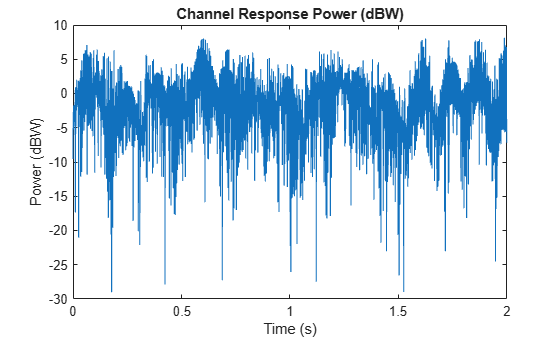Generate path gains for a 2-by-2 Rayleigh fading channel and examine the spatial correlation characteristics of the channel realization. Use the release object function to unlock the object to set the AntennaSelection property to 'Tx and Rx' and then confirm the unselected transmit-receive antenna pairs.

Create a 2-by-2 MIMO channel System object with two discrete paths and channel filtering disabled. Each path has different transmit and receive correlation matrices, specified by the TransmitCorrelationMatrix and ReceiveCorrelationMatrix properties.

mimoChan = comm.MIMOChannel( ...
'SampleRate',1000, ...
'PathDelays',[0 1e-3], ...
'AveragePathGains',[3 5], ...
'NormalizePathGains',false, ...
'MaximumDopplerShift',5, ...
'TransmitCorrelationMatrix',cat(3,eye(2),[1 0.1;0.1 1]), ...
'RandomStream','mt19937ar with seed', ...
'Seed',33, ...
'ChannelFiltering',false);

Generate channel response path gains using the MIMO channel object.

pathGains = mimoChan();

The transmit spatial correlation for the first discrete path at the first receive antenna is specified as an identity matrix in the TransmitCorrelationMatrix property. Confirm that the channel output pathGains exhibits the same statistical characteristics by using the corrcoef function to display the transmit spatial correlation for the first discrete path and the first receive antenna.

corrcoef(squeeze(pathGains(:,1,:,1)))
ans = 2×2 complex

1.0000 + 0.0000i  -0.3391 + 0.4285i
-0.3391 - 0.4285i   1.0000 + 0.0000i

The transmit spatial correlation for the second discrete path at the second receive antenna is specified as [1 0.1;0.1 1] in the TransmitCorrelationMatrix property. Confirm that the channel output pathGains exhibits the same statistical characteristics by using the corrcoef function to display the transmit spatial correlation for the second discrete path and the second receive antenna.

corrcoef(squeeze(pathGains(:,2,:,2)))
ans = 2×2 complex

1.0000 + 0.0000i  -0.8989 - 0.2663i
-0.8989 + 0.2663i   1.0000 + 0.0000i

The receive spatial correlation for the first discrete path at the second transmit antenna is specified as [1 0.2;0.2 1] in the ReceiveCorrelationMatrix property. Confirm that the channel output pathGains exhibits the same statistical characteristics by using the corrcoef function to display the receive spatial correlation for the first discrete path and the second transmit antenna.

corrcoef(squeeze(pathGains(:,1,2,:)))
ans = 2×2 complex

1.0000 + 0.0000i   0.9170 + 0.3141i
0.9170 - 0.3141i   1.0000 + 0.0000i

The receive spatial correlation for the second discrete path at the first transmit antenna is specified as an identity matrix in the ReceiveCorrelationMatrix property. Confirm that the channel output pathGains exhibits the same statistical characteristics by using the corrcoef function to display the receive spatial correlation for the second discrete path and the first transmit antenna.

corrcoef(squeeze(pathGains(:,2,1,:)))
ans = 2×2 complex

1.0000 + 0.0000i   0.9227 - 0.3435i
0.9227 + 0.3435i   1.0000 + 0.0000i

Create a frequency-selective MIMO channel, and then display its impulse and frequency responses.

Set the sample rate to 10 MHz. Specify path delays and gains using the extended vehicular A (EVA) channel parameters. Set the maximum Doppler shift to 70 Hz.

fs = 10e6;                                                % Hz
pathDelays = [0 30 150 310 370 710 1090 1730 2510]*1e-9;  % Seconds
avgPathGains = [0 -1.5 -1.4 -3.6 -0.6 -9.1 -7 -12 -16.9]; % dB
fD = 70;                                                  % Hz

Create a 2-by-2 MIMO channel System object, specifying the previously defined parameters and setting channel visualization to plot the impulse and frequency responses. By default, the plot displays the antenna pair corresponding to first transmit and receive antennas.

mimoChan = comm.MIMOChannel('SampleRate',fs, ...
'PathDelays',pathDelays, ...
'AveragePathGains',avgPathGains, ...
'MaximumDopplerShift',fD, ...
'Visualization','Impulse and frequency responses');

Generate random binary data, and then pass it through the MIMO channel. The impulse response plot enables you to easily identify the individual paths and their corresponding filter coefficients. The frequency response plot shows the frequency-selective nature of the EVA channel.

x = randi([0 1],1000,2);
y = mimoChan(x);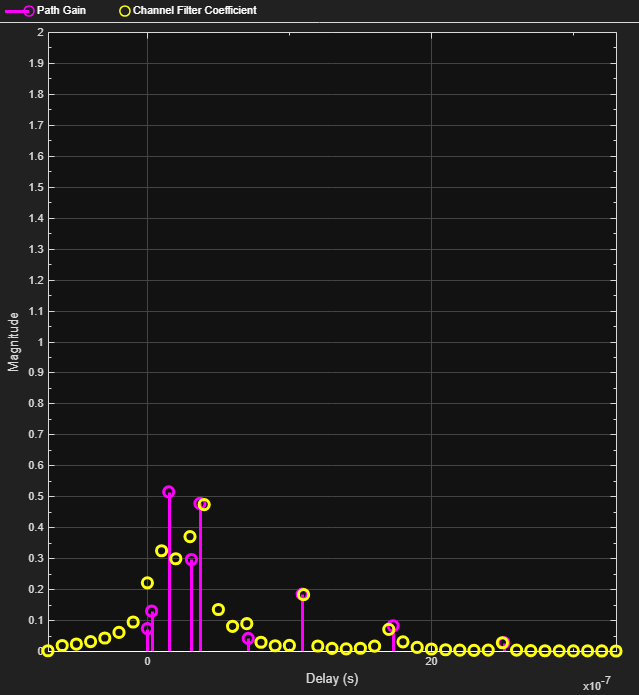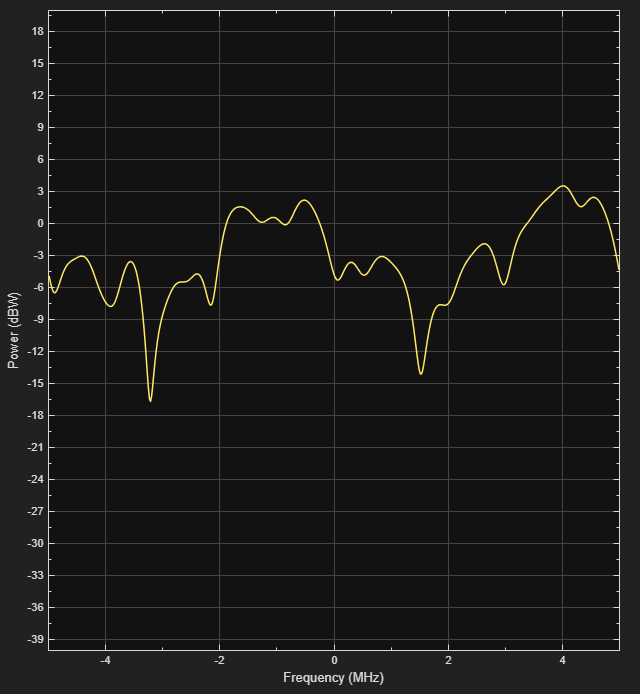To view the antenna pair corresponding to the second transmit and first receive antennas, release the MIMO channel System object, and then set its AntennaPairsToDisplay property to [2 1]. Because the AntennaPairsToDisplay property is nontunable, to change its value, you must release the System object.

release(mimoChan)
mimoChan.AntennaPairsToDisplay = [2 1];
y = mimoChan(x);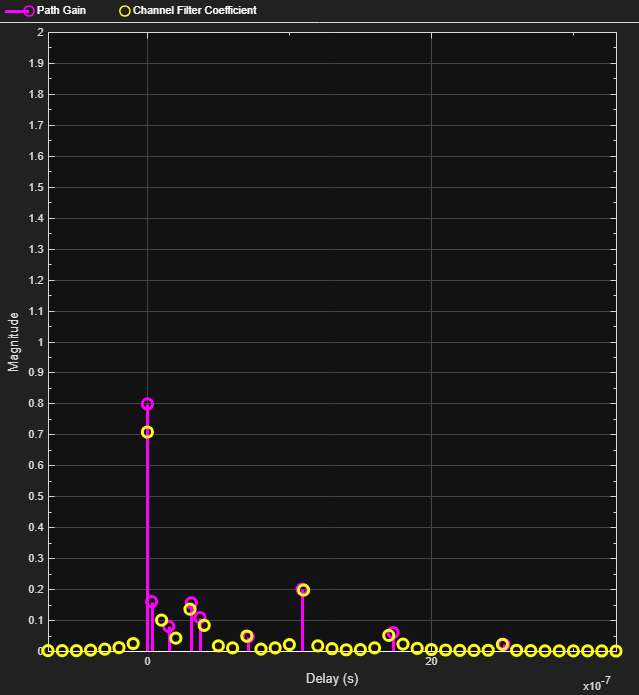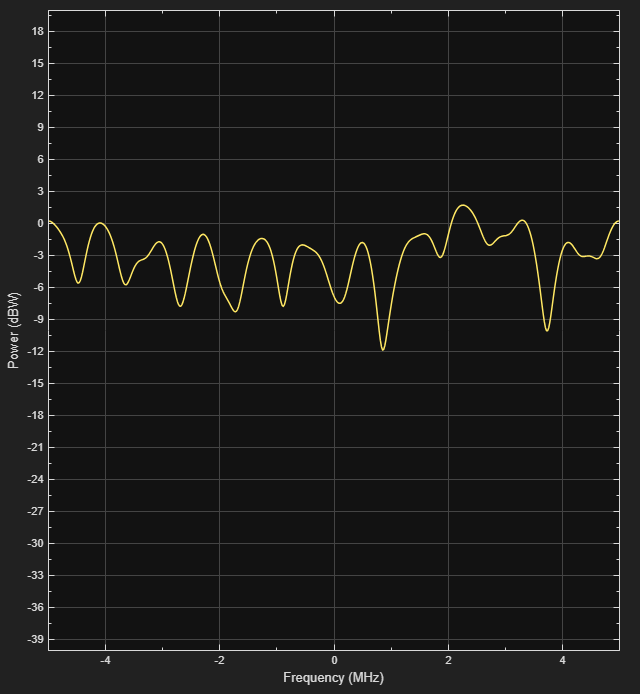Create and visualize the Doppler spectra of a MIMO channel that has two paths.

Construct a cell array of Doppler structures to be used in creating the channel. Set the Doppler spectrum of the first path to be bell shaped and the second path to be flat.

dp{1} = doppler('Bell');
dp{2} = doppler('Flat');

Create a 2-by-2 MIMO channel System object, specifying two paths and a maximum Doppler shift of 100 Hz, disabling the channel filtering, and enabling the visualization of the Doppler spectrum for the first Doppler path.

mimoChan = comm.MIMOChannel('SampleRate',1000, ...
'PathDelays',[0 0.002], ...
'AveragePathGains',[0 -3], ...
'MaximumDopplerShift',100, ...
'DopplerSpectrum',dp, ...
'ChannelFiltering',false, ...
'NumSamples',10000, ...
'Visualization','Doppler spectrum', ...
'PathsForDopplerDisplay',1);

Use the MIMO channel to generate the Doppler spectrum of the first path. Because the Doppler spectrum plot does not update until its buffer is filled, call the MIMO channel object multiple times to help improve the accuracy of the estimate. Observe that the spectrum has a bell shape and that its minimum and maximum frequencies fall within the limits set by the MaximumDopplerShift property.

for k = 1:25
mimoChan();
end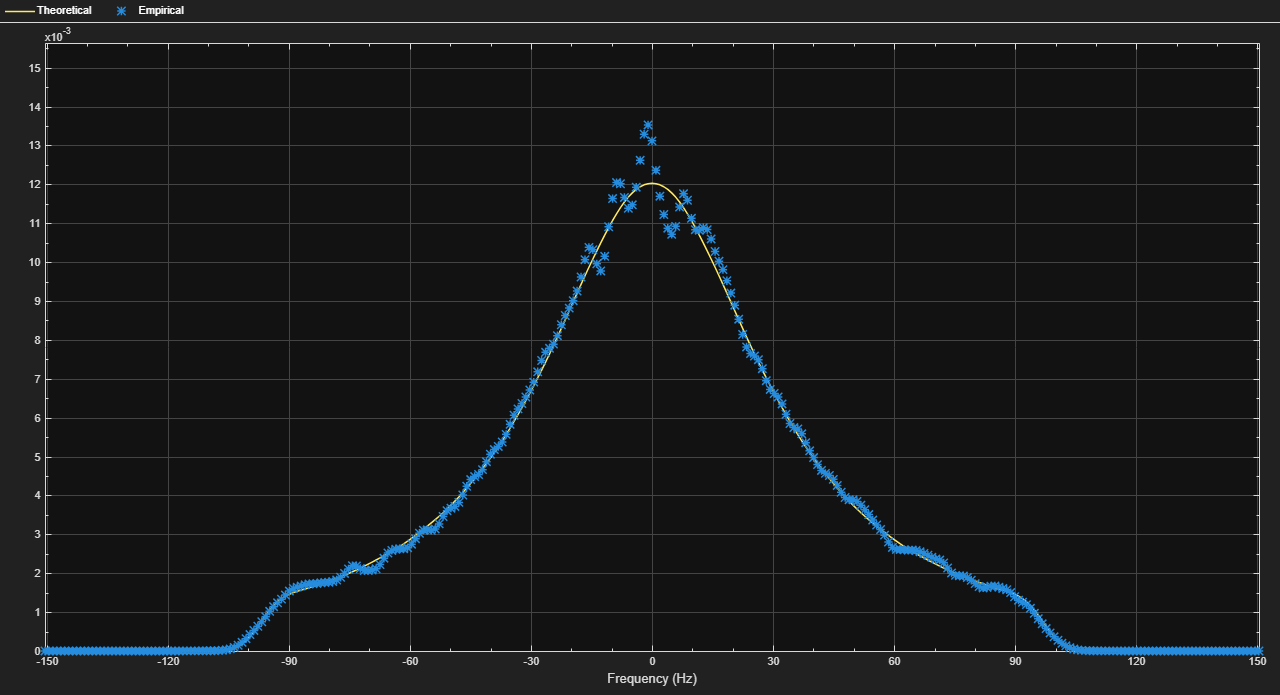Release the MIMO channel object, and set its PathsForDopplerDisplay property to display the second path. Because the PathsForDopplerDisplay property is nontunable, to change its value, you must release the System object. Call the object multiple times to display the Doppler spectrum of the second path. The results show that the spectrum is flat.

release(mimoChan)
mimoChan.PathsForDopplerDisplay = 2;
for k = 1:25
y = mimoChan();
end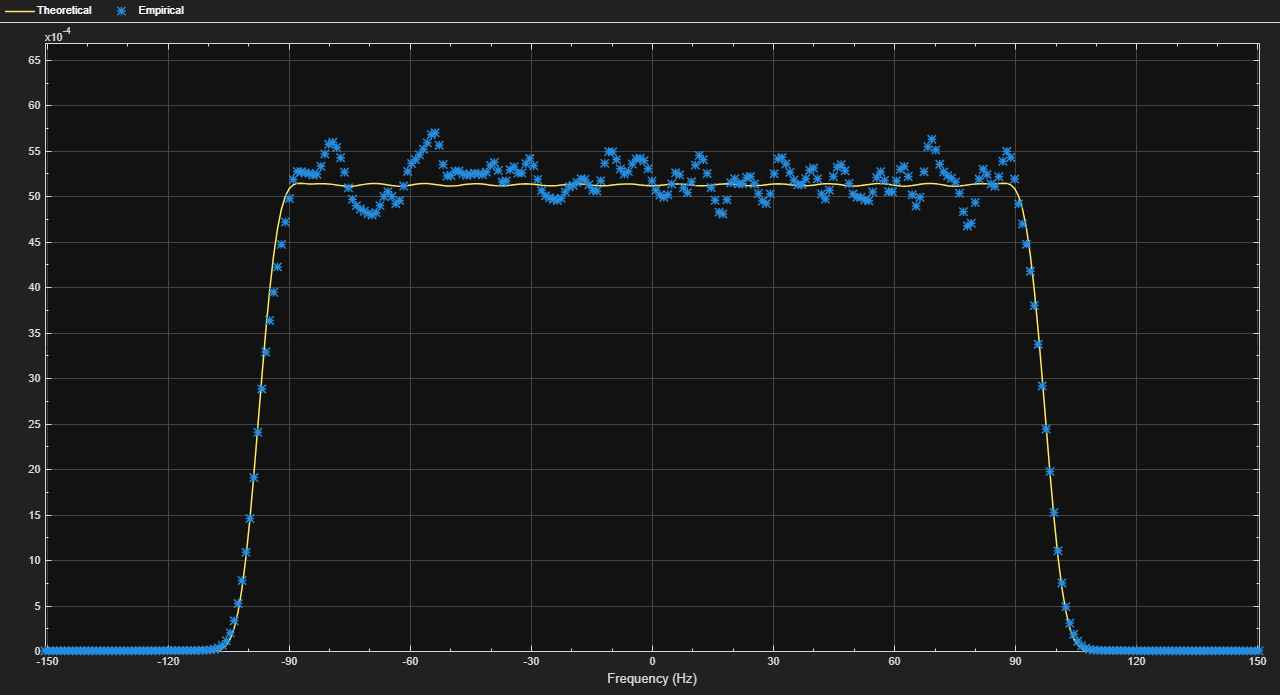Show that the channel state is maintained for discontinuous transmissions by using MIMO channel System objects configured to use the sum-of-sinusoids fading technique. Observe discontinuous channel response segments overlaid on a continuous channel response.

Set the channel properties.

fs = 1000;               % Sample rate (Hz)
pathDelays = [0 2.5e-3]; % Path delays (s)
pathPower = [0 -6];      % Path power (dB)
fD = 5;                  % Maximum Doppler shift (Hz)
ns = 1000;               % Number of samples
nsdel = 100;             % Number of samples for delayed paths

Define a continuous time span and three discontinuous time segments over which to plot and view the channel response. View a 1000-sample continuous channel response starting at time 0 and three 100-sample channel responses starting at times 0.1, 0.4, and 0.7 seconds, respectively.

to0 = 0.0;
to1 = 0.1;
to2 = 0.4;
to3 = 0.7;
t0 = (to0:ns-1)/fs;      % Transmission 0
t1 = to1+(0:nsdel-1)/fs; % Transmission 1
t2 = to2+(0:nsdel-1)/fs; % Transmission 2
t3 = to3+(0:nsdel-1)/fs; % Transmission 3

Create a flat-fading 2-by-2 MIMO channel System object, disabling channel filtering and specifying a 1000 Hz sampling rate, the sum-of-sinusoids fading technique, and the number of samples to view. Specify a seed value so that results can be repeated. Use the default InitialTime property setting so that the fading channel is simulated from time 0.

mimoChan1 = comm.MIMOChannel('SampleRate',fs, ...
'MaximumDopplerShift',fD, ...
'RandomStream','mt19937ar with seed', ...
'Seed',17, ...
'ChannelFiltering',false, ...
'NumSamples',ns);

Create a clone of the MIMO channel System object. Change the number of samples for the delayed paths and the source for the initial time so that you can specify the fading channel offset time as an input argument when calling the System object.

mimoChan2 = clone(mimoChan1);
mimoChan2.InitialTimeSource = 'Input port';
mimoChan2.NumSamples = nsdel;

Save the path gain output for the continuous channel response by using the mimoChan1 object and for the discontinuous delayed channel responses by using the mimoChan2 object with initial time offsets provided as input arguments.

pg0 = mimoChan1();
pg1 = mimoChan2(to1);
pg2 = mimoChan2(to2);
pg3 = mimoChan2(to3);

Compare the number of samples processed by the two channels by using the info method. The results show that mimoChan1 processed 1000 samples and that mimoChan2 processed only 300 samples.

G = info(mimoChan1);
H = info(mimoChan2);
[G.NumSamplesProcessed H.NumSamplesProcessed]
ans = 1×2

1000         300

Convert the path gains into decibels for the path corresponding to the first transmit and first receive antenna.

pathGain0 = 20*log10(abs(pg0(:,1,1,1)));
pathGain1 = 20*log10(abs(pg1(:,1,1,1)));
pathGain2 = 20*log10(abs(pg2(:,1,1,1)));
pathGain3 = 20*log10(abs(pg3(:,1,1,1)));

Plot the path gains for the continuous and discontinuous cases. The results show that the gains for the three segments match the gain for the continuous case. The alignment of the two shows that the sum-of-sinusoids technique is ideally suited to the simulation of packetized data because the channel characteristics are maintained even when data is not transmitted.

plot(t0,pathGain0,'r--')
hold on
plot(t1,pathGain1,'b')
plot(t2,pathGain2,'b')
plot(t3,pathGain3,'b')
grid
title('Continuous and Discontinuous Channel Response')
xlabel('Time (sec)')
ylabel('Path Gain (dB)')
legend('Continuous','Discontinuous','location','nw')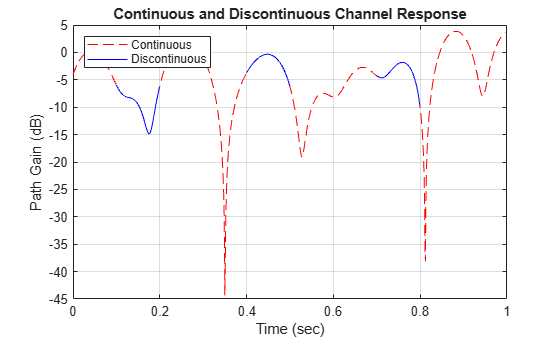Demonstrate the advantage of using the sum-of-sinusoids fading technique when simulating a channel with burst data.

Set the simulation parameters such that the sampling rate is 100 kHz, the total simulation time is 100 seconds, and the duty cycle for the burst data is 25%.

fs = 1e5;   % Hz
tsim = 100; % seconds
dutyCycle = 0.25;

Create a flat-fading 2-by-2 MIMO channel System object, specifying the sample rate and using the default filtered Gaussian noise technique.

fgn = comm.MIMOChannel('SampleRate',fs);

Create a similar MIMO channel System object, specifying the same sample rate as the previous MIMO channel object but using the sum-of-sinusoids technique. Additionally specify 48 sinusoids and for the fading process start times to be given as an input argument.

sos = comm.MIMOChannel('SampleRate',fs, ...
'NumSinusoids',48, ...
'InitialTimeSource','Input port');

Run a continuous sequence of random bits through the filtered Gaussian noise MIMO channel object. Use the tic and toc stopwatch timer functions to measure the execution time of the System object call.

tic
y = fgn(randi([0 1],fs*tsim,2));
tFGN = toc;

To transmit a data burst each second, pass random bits through the sum-of-sinusoids MIMO channel object by calling it inside of a for loop. Use the tic and toc stopwatch timer functions to measure the execution time.

tic
for k = 1:tsim
z = sos(randi([0 1],fs*dutyCycle,2),0.5+(k-1));
end
tSOS = toc;

Compare the ratio of the sum-of-sinusoids execution time to the filtered Gaussian noise execution time. The ratio is less than one, which indicates that the sum-of-sinusoids technique is faster than the filtered Gaussian noise technique.

tSOS/tFGN
ans = 0.3128

Using one MIMO channel System object™ and two identically configured channel filter System objects, switch a link-level simulation between 3-by-2 downlink and reciprocal 2-by-3 uplink signal transmissions.

Define system parameters.

modOrder = 256;        % Modulation order
Nant1 = 3;             % Number of 'transmit' antennas
Nant2 = 2;             % Number of 'receive' antennas
Rs = 1e6;              % Sample rate
pd = [0 1.5 2.3]*1e-6; % Path delays
frmLen = 1e3;          % Frame length

Create a MIMO channel System object™, configuring it for path gain generation by disabling channel filtering.

chan = comm.MIMOChannel( ...
'SampleRate',Rs, ...
'PathDelays',pd, ...
'AveragePathGains',[1.5 1.2 0.2], ...
'MaximumDopplerShift',300, ...
'SpatialCorrelationSpecification','none', ...
'NumTransmitAntennas',Nant1, ...
'ChannelFiltering',false, ...
'NumSamples',frmLen);

Create identical channel filter System objects for both transmission directions: one channel filter for the Nant1-by-Nant2 downlink channel (3 transmit antennas to 2 receive antennas) and a reciprocal channel filter for the Nant2-by-Nant1 uplink channel (2 transmit antennas to 3 receive antennas).

'SampleRate',Rs, ...
'PathDelays',pd);

Generate random path gains for one frame of the downlink 3-by-2 channel. Pass randomly generated 256-QAM signals through the 3-by-2 downlink channel.

x = qammod(randi([0 modOrder-1],frmLen,Nant1),modOrder);

Switch the link direction. Run the channel object to generate another frame of path gains, permuting its 3rd (Tx) and 4th (Rx) dimensions for the reciprocal uplink 2-by-3 channel. Pass randomly generated 256-QAM signals through the 2-by-3 reciprocal uplink channel.

pgUplink = permute(chan(),[1 2 4 3]);
x = qammod(randi([0 modOrder-1],frmLen,Nant2),modOrder);

Show the sizes of the downlink and uplink path gain arrays returned by the MIMI channel object as an ${\mathit{N}}_{\mathrm{S}}$-by-${\mathit{N}}_{\mathrm{P}}$-by-${\mathit{N}}_{\mathrm{T}}$-by-${\mathit{N}}_{\mathrm{R}}$ array.

• ${\mathit{N}}_{\mathrm{S}}$ is the number of samples.

• ${\mathit{N}}_{\mathrm{P}}$ is the number of path delays.

• ${\mathit{N}}_{\mathrm{T}}$ is the number of transmit antennas. Nant1 for downlink and Nant2 for uplink.

• ${\mathit{N}}_{\mathrm{R}}$ is the number of receive antennas. Nant2 for downlink and Nant1 for uplink.

ans = 1×4

1000           3           3           2

ans = 1×4

1000           3           2           3

Show the size of the channel output matrices returned by the MIMI channel object as an ${\mathit{N}}_{\mathrm{S}}$-by-${\mathit{N}}_{\mathrm{R}}$ matrix. ${\mathit{N}}_{\mathrm{S}}$ is the number of samples. ${\mathit{N}}_{\mathrm{R}}$ is the number of receive antennas.

size(yDL)
ans = 1×2

1000           2

size(yUL)
ans = 1×2

1000           3

Algorithms

expand all

The fading processing per link is described in Methodology for Simulating Multipath Fading Channels and assumes the same parameters for all (NT × NR) links of the MIMO channel. Each link comprises all multipaths for that link.

 Oestges, Claude, and Bruno Clerckx. MIMO Wireless Communications: From Real-World Propagation to Space-Time Code Design. 1st ed. Boston, MA: Elsevier, 2007.

 Correia, Luis M., and European Cooperation in the Field of Scientific and Technical Research (Organization), eds. Mobile Broadband Multimedia Networks: Techniques, Models and Tools for 4G. 1st ed. Amsterdam ; Boston: Elsevier/Academic Press, 2006.

 Kermoal, J.P., L. Schumacher, K.I. Pedersen, P.E. Mogensen, and F. Frederiksen. “A Stochastic MIMO Radio Channel Model with Experimental Validation.” IEEE Journal on Selected Areas in Communications 20, no. 6 (August 2002): 1211–26. https://doi.org/10.1109/JSAC.2002.801223.

 Jeruchim, Michel C., Philip Balaban, and K. Sam Shanmugan. Simulation of Communication Systems. Second edition. Boston, MA: Springer US, 2000.

 Patzold, M., Cheng-Xiang Wang, and B. Hogstad. “Two New Sum-of-Sinusoids-Based Methods for the Efficient Generation of Multiple Uncorrelated Rayleigh Fading Waveforms.” IEEE Transactions on Wireless Communications 8, no. 6 (June 2009): 3122–31. https://doi.org/10.1109/TWC.2009.080769.